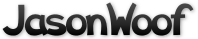Got questions, comments, patches, etc.? Contact Jason Woofenden
 author Joshua Grams Sun, 4 Mar 2007 13:42:54 +0000 (13:42 +0000) committer Joshua Grams Sun, 4 Mar 2007 13:42:54 +0000 (13:42 +0000)
 dust.c patch | blob | history

diff --git a/dust.c b/dust.c
index 7b62867..96a5805 100644 (file)
--- a/dust.c
+++ b/dust.c
@@ -13,6 +13,9 @@ struct dust_mote {

struct dust_mote motes[N_DUST_MOTES];

+// lower bound to ensure that we don't lose precision when wrapping.
+static float zero;
+
void
init_dust(void)
{
@@ -24,6 +27,8 @@ init_dust(void)
b = (MAX_DUST_DEPTH - motes[i].z) * 255.0 / MAX_DUST_DEPTH;
motes[i].color = SDL_MapRGB(surf_screen->format, b, b, b);
}
+
+       zero = pow(-10, ceil(log10(XSIZE)) - FLT_DIG);
}

void
@@ -32,34 +37,15 @@ move_dust(float ticks)
int i;
float xscroll = screendx * ticks;
float yscroll = screendy * ticks;
-
-       // Originally this code was much simpler, but it would crash sometimes
-       // because the floating point numbers wouldn't always round the same
-       // direction, and we'd ocanially try to draw off the screen.

for(i=0; i<N_DUST_MOTES; i++) {
motes[i].x -= xscroll / (1.3 + motes[i].z);
-               motes[i].y -= yscroll / (1.3 + motes[i].z);
-
-               if(motes[i].x < 0) {
-                       motes[i].x += XSIZE;
-               }
-               if(motes[i].x > (XSIZE - 0.000001)) {
-                       motes[i].x -= XSIZE;
-                       if(motes[i].x < 0) {
-                               motes[i].x = 0;
-                       }
-               }
+               if(motes[i].x >= XSIZE) motes[i].x -= XSIZE;
+               else if(motes[i].x < zero) motes[i].x += XSIZE;

-               if(motes[i].y < 0) {
-                       motes[i].y += YSIZE;
-               }
-               if(motes[i].y > (YSIZE - 0.000001)) {
-                       motes[i].y -= YSIZE;
-                       if(motes[i].y < 0) {
-                               motes[i].y = 0;
-                       }
-               }
+               motes[i].y -= yscroll / (1.3 + motes[i].z);
+               if(motes[i].y >= YSIZE) motes[i].y -= YSIZE;
+               else if(motes[i].y < zero) motes[i].y += YSIZE;
}
}